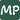# Sorting an Array of JavaScript Objects in a Specific Order

July 17, 2021

Sorting an array of objects in javascript is simple enough using the default sort() function for all arrays:

``````const arr = [
{
name: "Nina"
},
{
name: "Andre"
},
{
name: "Graham"
}
];
const sortedArr = arr.sort((a,b) => {
if ( a.name < b.name ){
return -1;
}
if ( a.name > b.name ){
return 1;
}
return 0;
});``````

And it is trivial enough to swap the sort order by switching the returns or the if statements above.

But what if you need to sort an array of objects in a specific, non-alphabetical order?

An example I came across was to transfer some SQL data for importing to a database and the transfer needed to occur in a table-dependent way, so as to not break the table constraints of importing foreign keys that didn’t exist yet.

``````// Defined sort order starting with the 'lowest' table in the SQL schema
const importOrder = ["Photo", "Address", "State", "Country"];

const tables = [
{
schema: []
},
{
name: "State",
schema: []
},
{
name: "Photo",
schema: []
},
{
name: "Country",
schema: []
}
];

const sortByObject = importOrder
.reduce((obj, item, index) => {
return {
...obj,
[item]: index,
};
}, {});

const customSort = tables.sort((a, b) => sortByObject[a.name] - sortByObject[b.name]);``````

So what’s going on here?

The key is the importOrder.reduce() function. This is transforming the importOrder array into an object that creates a numerical order for each item in the original import array:

``````// Output of sortByObjeect
{
Country: 3,
Photo: 0,
State: 2,
}``````

This then makes sorting the array much simpler by being able to directly look up an integer value for the sort position, which is what we are passing into the sort function of the tables array:

``````// Output of tables.sort()
[
{name: "Photo", schema: []},